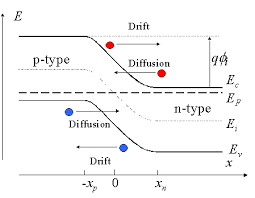# Valence band and Fermi level difference?

• I
nickhobbs
I was wondering what the difference was between the valence band and fermi level? How do we distinguish between the two?

## Answers and Replies

They are very different things. The valence band is the energy below which there are available states for electrons to occupy. Similarly the conduction band is the energy above which there are available states. Between these two is the energy gap, where there are no (or very few) available states. The Fermi level is the energy at which the probability of a state being occupied is 1/2. In the diagram below, for example, $E_V$ is the valence band, $E_C$ is the conduction band, and $E_F$ is the Fermi level.#### Attachments

•nickhobbs
nickhobbs
They are very different things. The valence band is the energy below which there are available states for electrons to occupy. Similarly the conduction band is the energy above which there are available states. Between these two is the energy gap, where there are no (or very few) available states. The Fermi level is the energy at which the probability of a state being occupied is 1/2. In the diagram below, for example, $E_V$ is the valence band, $E_C$ is the conduction band, and $E_F$ is the Fermi level.

View attachment 224005
Thanks for your response.

You say "The valence band is the energy below which there are available states for electrons to occupy" but my my Lecturer described the fermi level as the topmost filled level in the ground state of an N electron system, are these two different definitions?

•